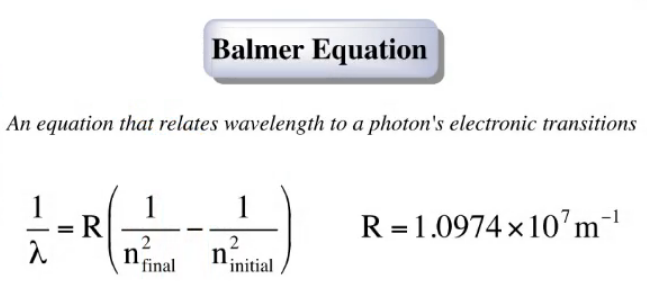Chemistry Practice Problems Bohr and Balmer Equations Practice Problems Solution: Does a photon of visible light (λ ≈ 400 to 700 nm)...

🤓 Based on our data, we think this question is relevant for Professor Decoste's class at UIUC.

# Solution: Does a photon of visible light (λ ≈ 400 to 700 nm) have sufficient energy to excite an electron in a hydrogen atom from the n = 1 to the n = 5 energy state? From the n = 2 to the n = 6 energy state?

###### Problem

Does a photon of visible light (λ ≈ 400 to 700 nm) have sufficient energy to excite an electron in a hydrogen atom from the n = 1 to the n = 5 energy state? From the n = 2 to the n = 6 energy state?

###### Solution

We use the Balmer Equation below to get the wavelength, needed to excite an electron with the given situations.We have to know that this equation is written to provide the wavelength for the energy emitted when an electron goes from a higher to a lower energy level.   To get the right sign, we just need to reverse the final and initial energy levels.View Complete Written Solution

Bohr and Balmer Equations

Bohr and Balmer Equations

#### Q. Calculate the longest and shortest wavelengths of light emitted by electrons in the hydrogen atom that begin in the n = 6 state and then fall to state...

Solved • Mon Oct 15 2018 14:40:48 GMT-0400 (EDT)

Bohr and Balmer Equations

#### Q. Calculate the wavelength of light emitted when the following transition occur in the hydrogen atom. n = 4 → n = 3What type of electromagnetic radiatio...

Solved • Mon Oct 15 2018 14:29:57 GMT-0400 (EDT)

Bohr and Balmer Equations

#### Q. Calculate the wavelength of light produced if an electron moves from n = 5 state to n = 3 state of an electron in a hydrogen atom.Express your answer ...

Solved • Fri Oct 05 2018 13:00:11 GMT-0400 (EDT)

Bohr and Balmer Equations

#### Q. An electron in the n = 5 level of an H atom emits a photon of wavelength 434.17 nm. To what energy level does the electron move?

Solved • Mon Sep 24 2018 18:36:15 GMT-0400 (EDT)